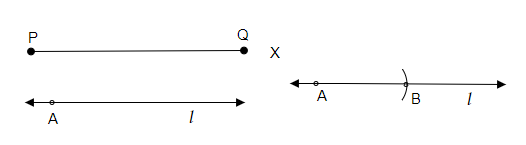# Draw any line segment $\overline{\mathrm{PQ}}$. Without measuring $\overline{\mathrm{PQ}}$, construct a copy of $\overline{\mathrm{PQ}}$.

To do:

We have to draw any line segment $\overline{PQ}$ and without measuring $\overline{PQ}$, we have to construct a copy of $\overline{PQ}$.

Solution:Steps of construction:

(i) First let us draw any line segment $\overline{PQ}$.

(ii) Then by pointing the pointer of compasses on point P let us adjust the compasses to point Q.

(iii) Let us draw a line 'l' and mark a point A on it.

(iv) Now by placing the pointer of the compasses on point A draw an arc on the line 'l' and mark it as point B.

(v) Therefore, the required $\overline{PQ}$  is formed.

Updated on: 10-Oct-2022

41 Views